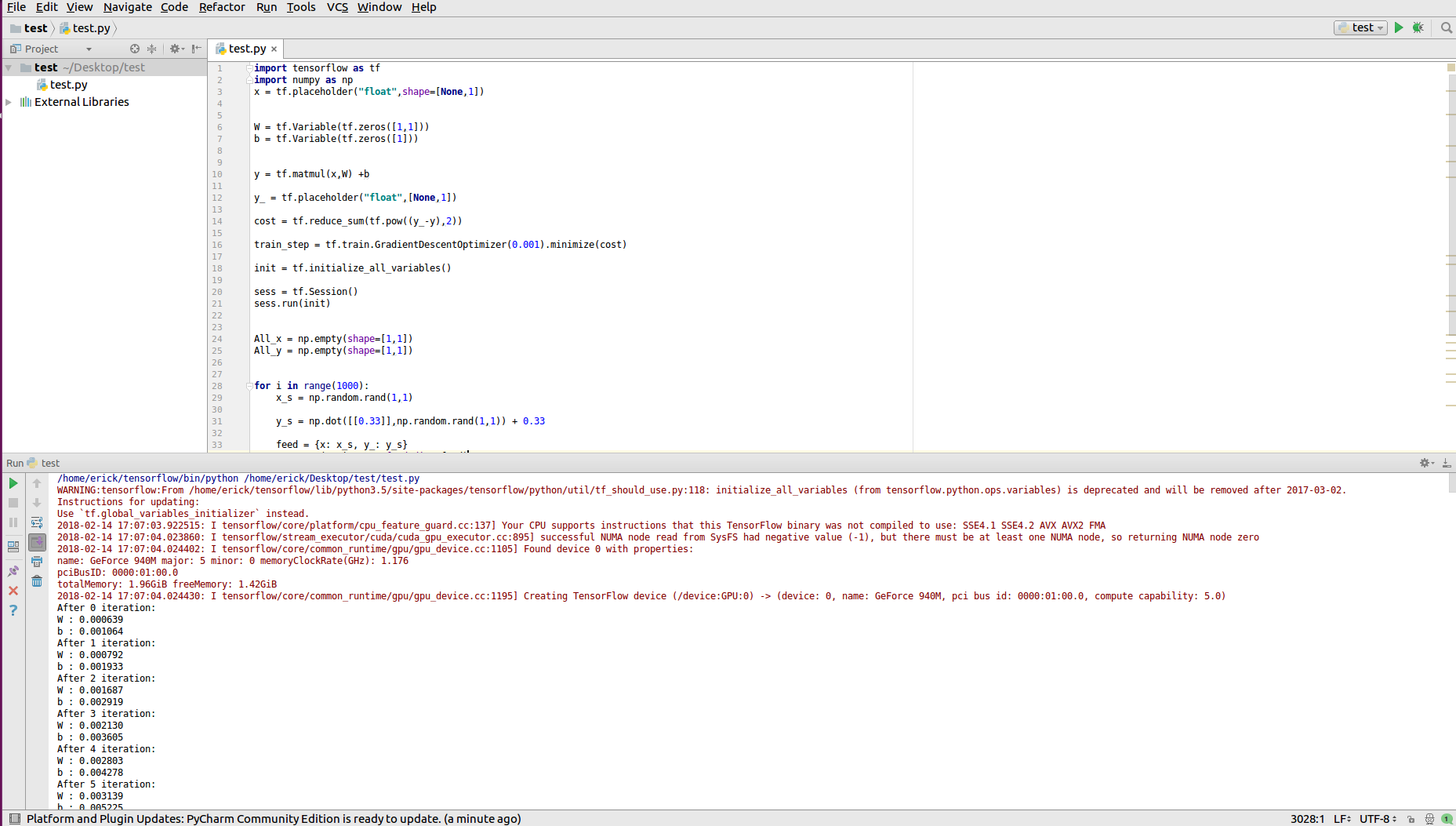Ubuntu + CUDA9.0 + tensorflow-gpu 安装过程

简介：

tensorflow支持CUDA9.0和cuDNN7.0，因此本教程是在该版本基础上进行安装的。我的电脑CPU是Intel core i7 4710MQ，GPU是GTX940M，请大家在安装前自己检查下硬件是否符合要求。

系统说明：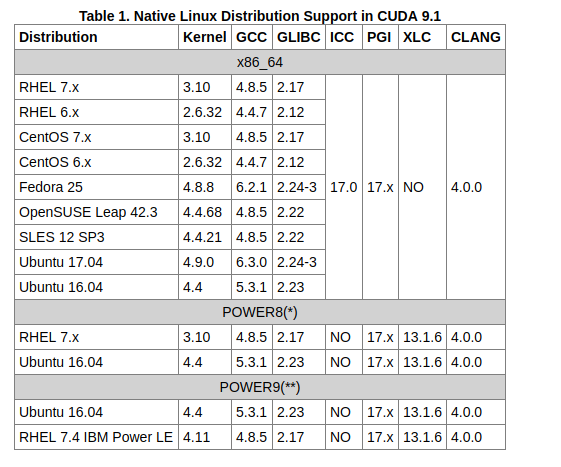安装CUDA® Toolkit 9.0.1. sudo dpkg -i cuda-repo-ubuntu1604-9-0-local_9.0.176-1_amd64.deb
2. sudo apt-key add /var/cuda-repo-9-0-local/7fa2af80.pub
3. sudo apt-get update
4. sudo apt-get install cuda

1. export PATH=/usr/local/cuda-9.0/bin${PATH:+:${PATH}}
2. 如果是64位系统，输入：
export LD_LIBRARY_PATH=/usr/local/cuda-9.0/lib64${LD_LIBRARY_PATH:+:${LD_LIBRARY_PATH}}
3. 如果是32位系统，输入：
export LD_LIBRARY_PATH=/usr/local/cuda-9.0/lib${LD_LIBRARY_PATH:+:${LD_LIBRARY_PATH}}

安装cuDNN v7.0.

**cuDNN v7.0.**下载地址：https://developer.nvidia.com/cudnn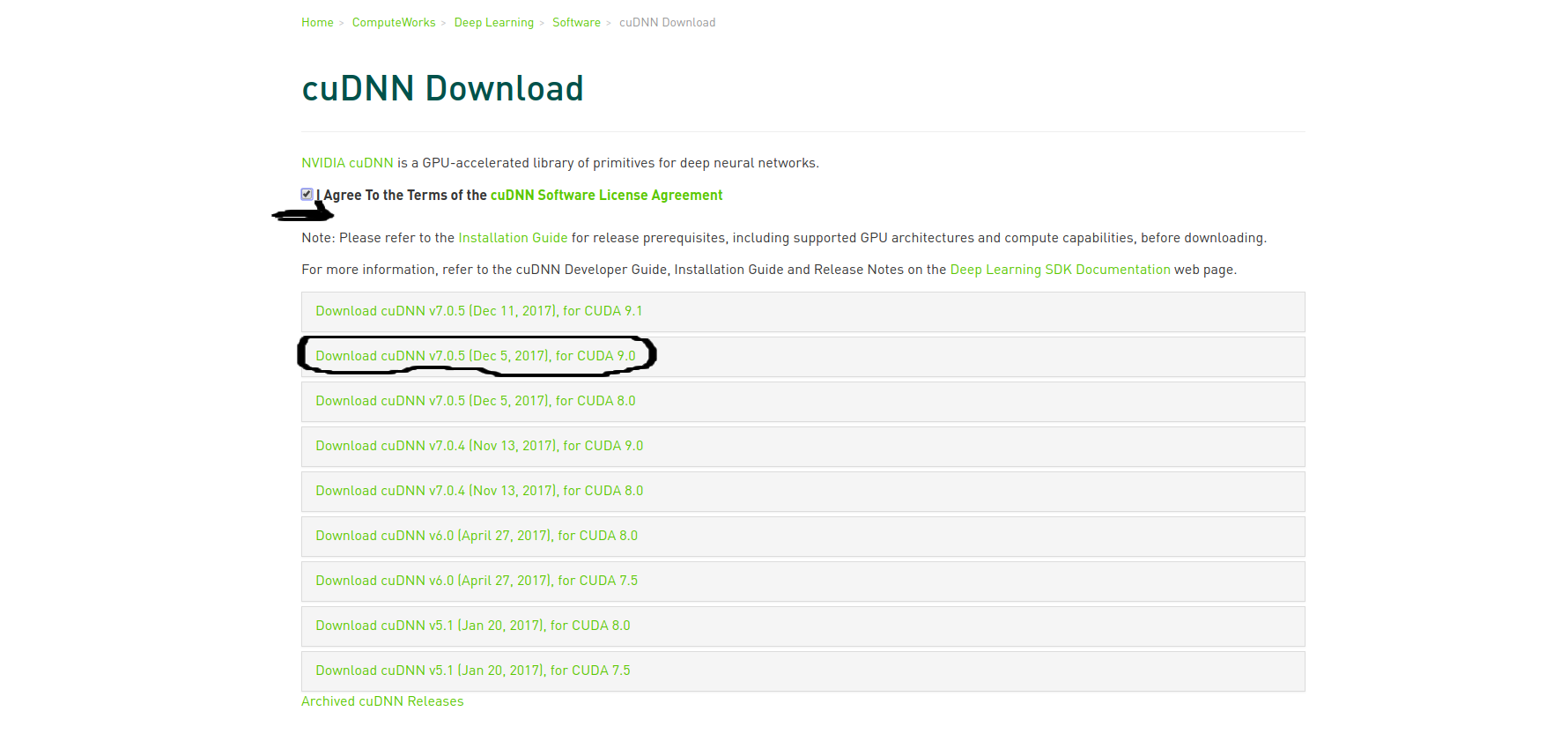sudo dpkg -i libcudnn7_7.0.5.15-1+cuda9.0_amd64.deb

安装Tensorflow-GPU版本

GPU相比于CPU，再运算速度上有很大的优势，这也是本文推荐安装GPU版本的原因。

Tensorflow总共有4中安装方式，在这里，我们使用Google官方推荐的安装方式：Virtualenv，创建一个虚拟Python开发环境。

1. 安装pip和Virtualenv
打开终端；
如果使用Python 2.7的版本，输入sudo apt-get install python-pip python-dev python-virtualenv
如果使用Python 3.x的版本，输入sudo apt-get install python3-pip python3-dev python-virtualenv
两者选择一个就行，个人推荐Python 3.x版本

2. 创造一个虚拟的Python开发环境：
第一步如果选择Python 2.7版本，终端输入：virtualenv --system-site-packages ~/tensorflow
第一步如果选择Python 3.x版本，终端输入：virtualenv --system-site-packages -p python3 ~/tensorflow
注意，~/tensorflow是自己选择的位置并创建的目录。大家可以自行选择其他的位置和命名。本教程创建完成之后，会在用户家目录下看到多出的tensorflow文件夹：3. 激活虚拟环境：
如果你的终端是bash, sh, ksh, zsh中的一个，那么输入：source ~/tensorflow/bin/activate
如果你的终端是csh,tcsh，那么输入：source ~/tensorflow/bin/activate.csh
如果你不知道的话。。。。那就输入第一个吧，Ubuntu默认是第一个。。。。。
之后，会再终端中看到：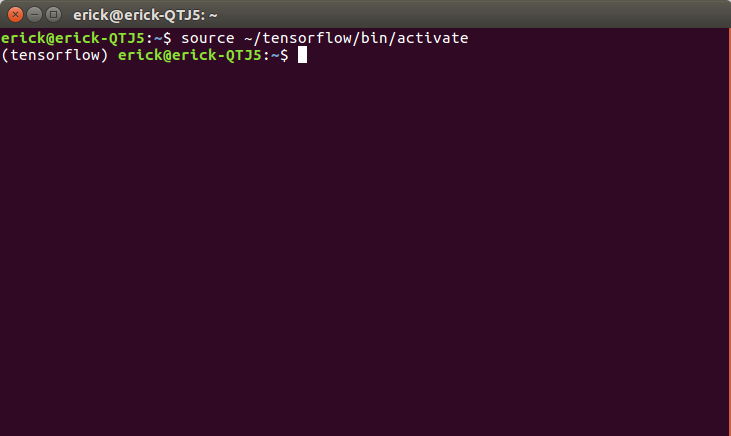4. 保证pip的版本不低于8.1，再虚拟环境中输入：easy_install -U pip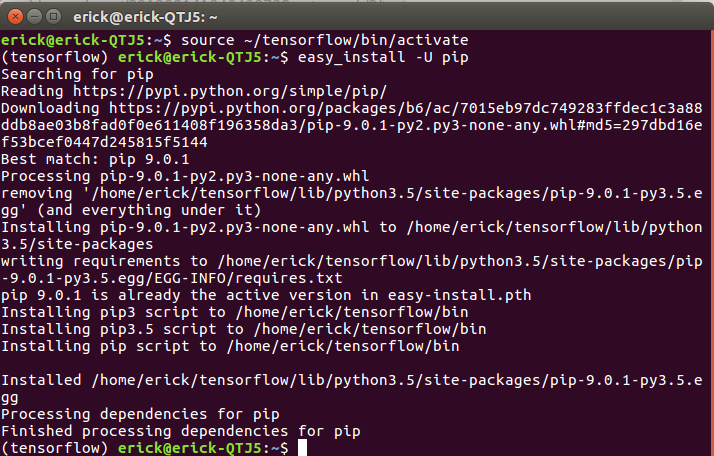5. 安装tersorflow-gpu版本：
Python 2.7版本：pip install --upgrade tensorflow-gpu
Python 3.x版本：pip3 install --upgrade tensorflow-gpu
经过这一步骤之后，tensorflow就安装完成了。

如果安装速度慢，且没法fanqiang的话，可以使用清华的源
pip install -i https://pypi.tuna.tsinghua.edu.cn/simple tensorflow-gpu
pip3 install -i https://pypi.tuna.tsinghua.edu.cn/simple tensorflow-gpu

6. 以后，如果需要使用tenssorflow，则打开终端输入：source ~/tensorflow/bin/activate,如果关闭虚拟环境，输入deactivate即可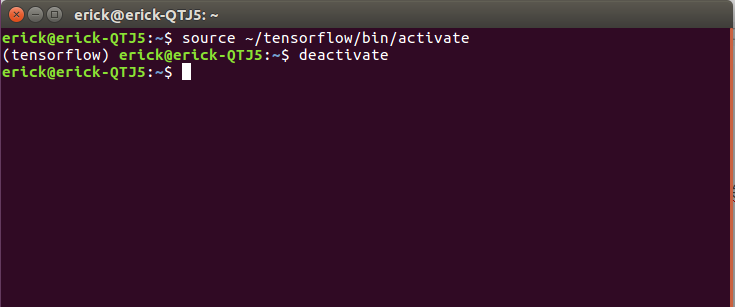7. 有些IDE 会自动检测tensorflow创建的虚拟环境，不必在终端中单独开启或者关闭，比如pycharm等。

import tensorflow as tf
import numpy as np
x = tf.placeholder("float",shape=[None,1])

W = tf.Variable(tf.zeros([1,1]))
b = tf.Variable(tf.zeros())

y = tf.matmul(x,W) +b

y_ = tf.placeholder("float",[None,1])

cost = tf.reduce_sum(tf.pow((y_-y),2))

init = tf.initialize_all_variables()

sess = tf.Session()
sess.run(init)

All_x = np.empty(shape=[1,1])
All_y = np.empty(shape=[1,1])

for i in range(1000):
x_s = np.random.rand(1,1)

y_s = np.dot([[0.33]],np.random.rand(1,1)) + 0.33

feed = {x: x_s, y_: y_s}
sess.run(train_step,feed_dict=feed)
print("After %d iteration:"%i)
print("W : %f"%sess.run(W))
print("b : %f"%sess.run(b))

All_x = np.concatenate((All_x,x_s))
All_y = np.concatenate((All_y,y_s))

print(All_x)
print(All_y)